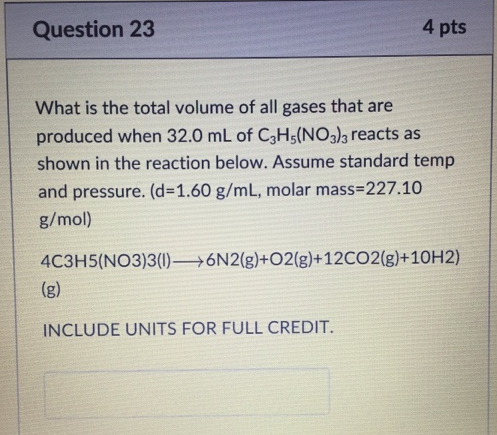Chemistry Practice Problems Gas Stoichiometry Practice Problems Solution: What is the total volume of all gases that are pro...

🤓 Based on our data, we think this question is relevant for Professor Tsitovich's class at GSU.

# Solution: What is the total volume of all gases that are produced when 32.0 mL of C3H5(NO3)3 reacts as shown in the reaction below. Assume standard temp and pressure. (d = 1.60 g/mL, molar mass 227.10 g/mol) 4C3H5(NO3)3 (I) → 6N2(g) + O2(g) + 12CO2(g) + 10H2 (g).

###### Problem

What is the total volume of all gases that are produced when 32.0 mL of C3H5(NO3)3 reacts as shown in the reaction below. Assume standard temp and pressure. (d = 1.60 g/mL, molar mass 227.10 g/mol) 4C3H5(NO3)3 (I) → 6N2(g) + O2(g) + 12CO2(g) + 10H2 (g).View Complete Written Solution

Gas Stoichiometry

Gas Stoichiometry

#### Q. Hydrogen and iodine gases react to form hydrogen iodide gas as follows: H2 (g) + I2 (g) → 2HI (g) At a certain temperature and pressure 1.4 L of H 2 r...

Solved • Wed Jul 04 2018 13:50:12 GMT-0400 (EDT)

Gas Stoichiometry

#### Q. Chlorine gas is collected from the following reaction of MnO2(s) with HCl:MnO2(s) + 4HCl(aq) → MnCl2(aq) + Cl2(g) + 2H2O(l) Calculate the volume of HC...

Solved • Tue Jun 19 2018 15:19:46 GMT-0400 (EDT)

Gas Stoichiometry

#### Q. Chlorine gas reacts with fluorine gas to form chlorine trifluoride. Cl2 (g) + 3F2 (g) → 2 ClF3 (g) A 2.00 L reaction vessel, initially at 298 K, conta...

Solved • Tue Jun 19 2018 15:01:51 GMT-0400 (EDT)

Gas Stoichiometry

#### Q. Ammonium carbonate decomposes upon heating according to the following balanced equation: (NH4)2CO3 (s) → 2 NH3 (g) + CO2 (g) + H2O (g) Calculate the t...

Solved • Tue Jun 19 2018 14:58:19 GMT-0400 (EDT)